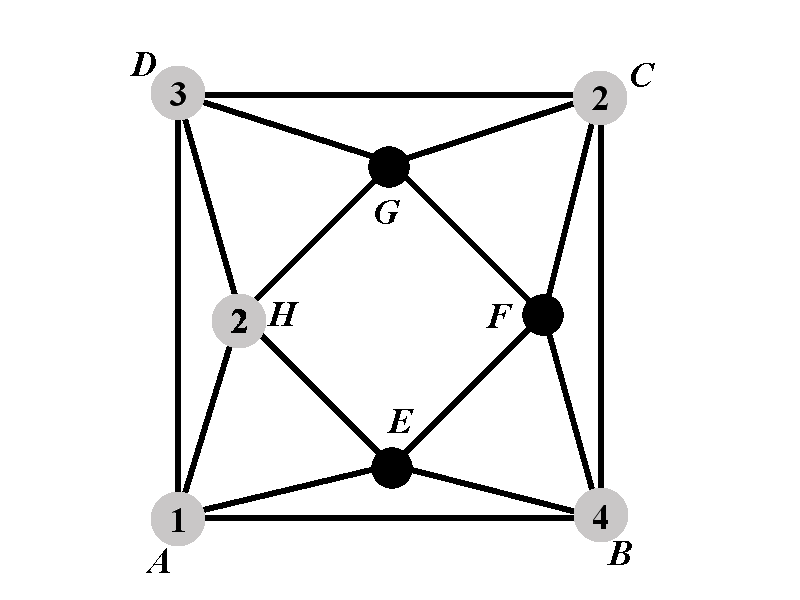Back to: Chromatic Polynomial Case $$2.2^{\circ}$$
##### Case 2.2.1: Calculation of $$P_{2.2.1^{\circ}}(k)$$Observe that the vertex $$G$$ cannot have a color $$2$$ or $$3$$. We will consider 3 sub-cases. Sub-case 2.2.1.1 is in which the vertex $$G$$ has color $$1$$. Sub-case 2.2.1.2 is the sub-case in which $$G$$ has color $$4$$, and sub-case 2.2.1.3 is the sub-case in which $$G$$ has a color outside of the set $$\{1,2,3,4\}$$.

Let us introduce the following polynomials that correspond to sub-cases 2.2.1.1, 2.2.1.2, and 2.2.1.3.

• Denote by $$P_{2.2.1.1^{\circ}}(k)$$ the number of proper colorings of $$\Gamma$$ in $$k$$ colors such that the vertices $$A$$, $$B$$, $$C$$, $$D$$, $$G$$, and $$H$$ have the colors $$1$$, $$4$$, $$2$$, $$3$$, $$1$$, and $$2$$, respectively.

• Denote by $$P_{2.2.1.2^{\circ}}(k)$$ the number of proper colorings of $$\Gamma$$ in $$k$$ colors such that the vertices $$A$$, $$B$$, $$C$$, $$D$$, $$G$$, and $$H$$ have the colors $$1$$, $$4$$, $$2$$, $$3$$, $$4$$, and $$2$$, respectively.

• Denote by $$P_{2.2.1.3^{\circ}}(k)$$ the number of proper colorings of $$\Gamma$$ in $$k$$ colors such that the vertices $$A$$, $$B$$, $$C$$, $$D$$, $$G$$, and $$H$$ have the colors $$1$$, $$4$$, $$2$$, $$3$$, $$5$$, and $$2$$, respectively.

The polynomial $$P_{2.2.1^{\circ}}(k)$$ can be expressed in terms of these newly introduced polynomials as $P_{2.2.1^{\circ}}(k)=P_{2.2.1.1^{\circ}}(k)+P_{2.2.1.2^{\circ}}(k)+(k-4)P_{2.2.1.3^{\circ}}(k).$ The last polynomial is multiplied by $$(k-4)$$ because if the vertex $$G$$ does not have any of the colors from $$\{1,2,3,4\}$$, then the number of available colors for $$G$$ is $$k-4$$.

Therefore the polynomial $$P_{2.2.1^{\circ}}(k)$$ satisfies \begin{eqnarray*} P_{2.2.1^{\circ}}(k)&=&P_{2.2.1.1^{\circ}}(k)+P_{2.2.1.2^{\circ}}(k)+(k-4)P_{2.2.1.3^{\circ}}(k)\newline &=&(k-3)(k-4)+(k-3)^2+(k-4)\left( k-3+(k-4)^2\right)\newline &=&k^3-9k^2+28k-31. \end{eqnarray*}